## 2.8Flow in a volume

We presented conservation of momentum, Eq. (2.19 ), in terms of the material derivative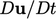. To solve the equation in this form, would require a method that tracks particles of ﬂuid as they move around.

Generally it is easier to solve the equation by ﬁxing the volume of space and solving for the ﬂuid motion through it. To enable this, we replace the material derivative for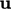using the following expression: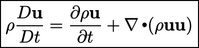(2.26)
Eq. (2.26 ) is derived from the material derivative Eq. (2.14 ) by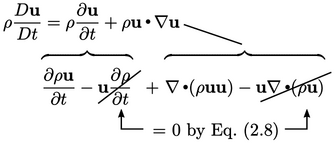Conservation of momentum can then be written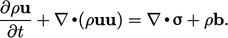(2.27)
A term of the form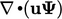or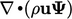represents the bulk motion of the ﬂuid at velocity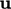, which transports the property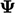(a tensor of any rank) by advection6. For example, if the propertyis temperature, then advection will transport heat from one region of the ﬂow domain to another.

Advection contains a divergence derivative so represents a ﬂux across a surface per unit volume. The example in Eq. (2.27 ) represents the ﬂux of momentum, where the advected property is itself(or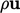, depending whether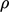is associated with bulk ﬂow or the advected property).

### Outer product of two vectors

The advection term in Eq. (2.27) includes a product of two vectors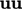. This is the outer product of two vectors7 which produces a tensor by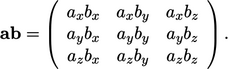(2.28)

### Inner product of two tensors

The inner product of two tensors, e.g.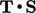produces a tensor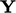where the components are (replacing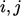with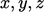)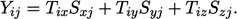(2.29)

### Identity tensor

The identity tensor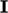is the tensor equivalent of unity (one) such that for any tensor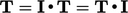,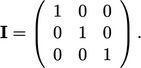(2.30)

6The term “convection” is sometimes used, but it has conﬂicting meanings, so we avoid it here.
7sometimes written “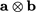”, but we write it as a product with no symbol, similar to a scalar multiplication.

Notes on CFD: General Principles - 2.8 Flow in a volume